Power BI Skills - Thinking of Data in Tables - Excelerator BI

# Power BI Skills – Thinking of Data in Tables

Level: Beginners

One very important concept that Excel users often struggle with when starting on their Power BI and Power Pivot journey is that they need to learn to think about data in tables rather than thinking about data in cells.  In the spreadsheet world every cell can be a different formula or value, but in the Power BI and Power Pivot worlds, it doesn’t work this way.

## The Excel Way

The Excel way is very simple.  You load data into the spreadsheet – each cell in the spreadsheet contains one data element (one scalar value).  From there you can write a formula in a single cell (see cell E2 below).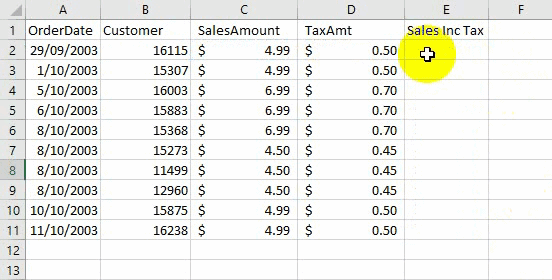If you want the same formula to appear many times in your spreadsheet, you copy the formula down the page. The key point here is that every cell in the above example is either a scalar value or an individual formula that returns a scalar value. That is how traditional Excel works and that is why us Excel folk are comfortable thinking about data in this way.

### But What About Excel Tables I Hear You Say?

Yes, good point.  Sometime in the past Excel was given a table object as part of it suite of tools.  Many Excel users don’t know about these and hence don’t use them.  Excel tables solve quite a few issues compared with Excel cell ranges including:

• allowing you to write a single formula, and have that formula apply to the entire column in the table
• extending the formulas in the table when there are new rows added to the table
• extending a reference (such as a pivot table source reference) so that it will point to a table (that can change size and shape) rather than pointing to a static range of cells.

Below I convert the same Excel range from the first image into an Excel table.  Note how I now only need to add a single formula to create “Sales Inc Tax”.  The single formula now applies to the entire table.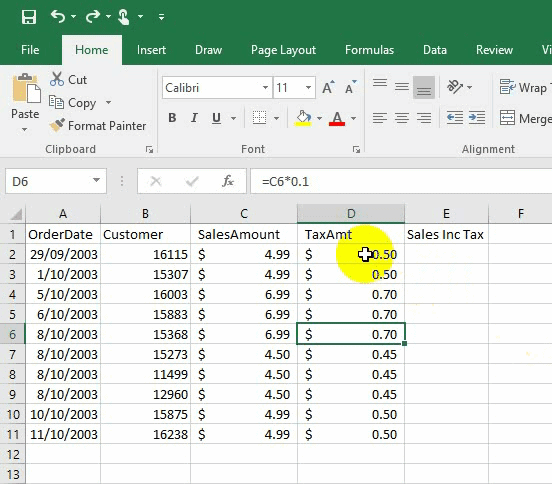When you have an Excel table like the one above, you can then start to refer to the entire table or columns in other formulas in your spreadsheet.  See in the image below how the formula in cell G3 refers to a single column in the table.  As the table grows, the formula doesn’t need to be changed – it just works.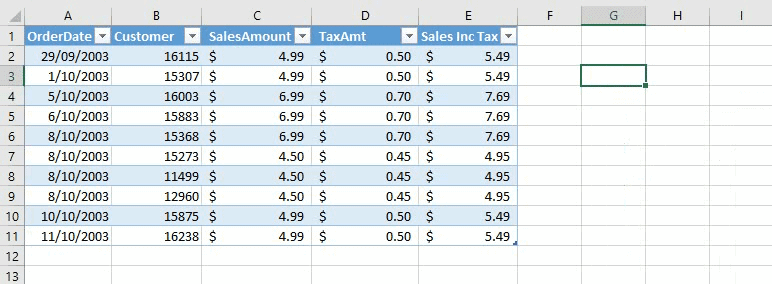This use of tables in Excel as shown above is very similar to how it works in Power Pivot and Power BI.  You no longer think about clusters of cells and instead have to consider the entire table or column.

## Step 1: Thinking of Data in Tables in Power Pivot

The key things you need to know as you learn to think of data in tables in Power Pivot are:

1. Measures are written to operate over the entire data model.  You can (and normally do) get a different answer to the same formula depending on what filtering is currently applied to the columns, tables and visuals in your data model/report.
2. You can’t directly reference rows in a table. The only way to reference specific row(s) is to first “filter” the table so that the rows you want to access are “visible” (and all the rows you don’t want are “not visible”).  After the filter is applied, then the measure or calculated column (mentioned in point 1 above) will work for the filtered copy of the table (it is a little more complicated than that for calculated columns but that is beyond the scope of this article).
3. You need to learn to “imagine” what the table looks like with filters applied.  The reason you need to learn this skill is because many of the tables you will use in DAX formulas are ‘virtual tables’, that is the table is never materialised in a way that you can see it.  You need to learn to “imagine” what the table looks like in its filtered state.  I wrote a blog post at powerpivotpro.com that explains how you can use Power BI to physically materialise these virtual tables into temporary “test tables” to help you get started on your journey of being able to visualise what is happening.  Once you get the hang of it you will only need to materialise a table when you can’t work out why your formula isn’t working or if there is a specific question that is too hard to answer without looking (like question 5 that you will see later in this post).

### Here is some imagination practice for you

Imagine you have a calendar table containing every date from 1 Jan 2010 to 31 Dec 2016 as well as columns for [Day Name], [Month Name] and [Year].  It would look something like this.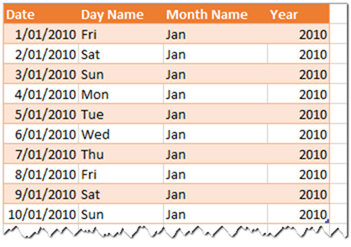This table of course would have more than 2,500 rows.  Picture this table in your mind (eg stop looking at the image above).  Now imagine what would happen if you applied a filter on the Year column to be equal to 2012 and another filter on the Month Name column to be Feb.  Once you have applied these filters in your mind, then answer the following questions by referring to your imaginary table in your mind.

1. What would the table look like now?
2. How many rows are there visible in this table with these 2 filters applied?

## Step 2: Thinking of Data in Columns

As well as mastering tables, you need to master columns.  Here are some additional questions for your imaginary table – this time the questions are about columns.

1. How many unique values now appear in the Month Name column?
2. How many unique values now appear in the Day Name column?
3. What is the minimum date that exists in the Date column?
4. What is the maximum date that exists in the Date column?
5. What is the Day Name for the last date in this filtered table?

The answers are are at the bottom of the page.  But do yourself a favour and answer each question yourself without cheating – this will help you understand how skilled you are with thinking of data in tables and columns using your mind’s eye.  In fact I don’t expect you to be able to answer number 5 without doing some research.

The reason you need to think about columns of data are two fold.

• Many of your formulas will operate over single or multiple columns.
• Power Pivot is a columnar database and it is optimised for working with columns.

As a general rule, it is more efficient to apply filters to individual columns one at a time rather than apply filters to multiple columns at the same time.  Consider the following two measures.

```Count of days inefficient =
CALCULATE(
COUNTROWS('Calendar'),
FILTER('Calendar','Calendar'[Year] = 2012 && 'Calendar'[Month Name] = “Feb”)
)```
```Count of days efficient =
CALCULATE(
COUNTROWS('Calendar'),
FILTER('Calendar','Calendar'[Year] = 2012),
FILTER('Calendar','Calendar'[Month Name] = “Feb”)
)```

The second formula is much more efficient because there are 2 separate filters being applied to 2 separate columns and they are applied one at a time (in a logical AND).  This is much more efficient than asking Power Pivot to consider both columns at the same time.

Note the FILTER functions in these two measures above all return a filtered copy of the calendar table.  You can’t see the filter copy of the table and that can make it hard to understand what is happening.  But if you learn to imagine what the tables look like in their filtered state you will be well on the way to becoming a DAX super star.## Step 3: Thinking of the Entire Data Model

The final thing you need to learn is that the entire data model operates as an enclosed ecosystem.  In the data model shown below, there are 4 lookup tables at the top of the image and 2 data tables at the bottom (from Adventure Works).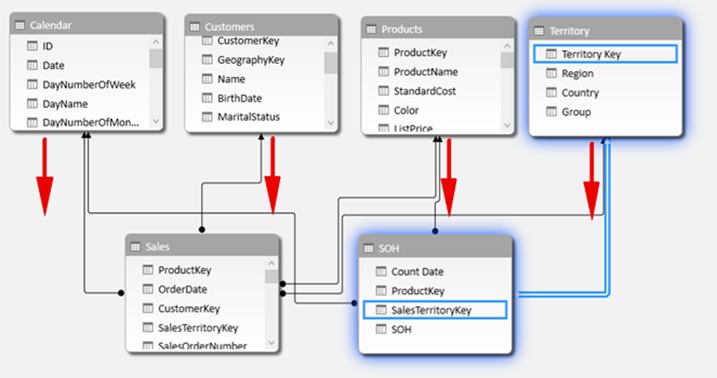All 6 of these tables operate as a single system.  Filters applied to the lookup tables propagate downhill from the top (one side of the relationship) to the bottom (many side of the relationship).  Filters do not automatically propagate from the bottom to the top.  So once you learn to think about how filtering will affect a single table, you then need to take a further step to imagine how the filters will propagate to all other tables in the data model.## Answers to the Quizzes Above

### Tables

1. What would the table look like now?  It would still have 4 columns but now it has only 29 rows.
2. How many rows are there visible in this table with the filters applied?  29.

### Columns

1. How many unique values appear in the Month Name column?  1 – Feb.
2. How many unique values appear in the Day Name column? 7, the days from Sun to Sat
3. What is the minimum date that exists in the Date column?   1 Feb 2012
4. What is the maximum date that exists in the Date column?  29 Feb 2012
5. What is the Day Name for the last date in this filtered table?  It is Wednesday, but this is a very hard question and too difficult to answer without either materialising the table or doing some research to check the day of week for the 29th Feb 2012.

### 11 thoughts on “Power BI Skills – Thinking of Data in Tables”

1.Hi Matt,
Great article. You have written: “As a general rule, it is more efficient to apply filters to individual columns one at a time rather than apply filters to multiple columns at the same time.”
Is the order of filters important? Does it matter? For example filter year first and then month or filter month and then year? Does it make any difference for Power Pivot engine?

1.The execution by the engine may be different to what you actually write. I am pretty confident there is no meaningful difference in the order you write the filters under average circumstances. One possible exception could be when you have nested filters. Ie instead of multiple column filters that use the same calculate, there are 2 calculate functions with inner and outer filters. Generally low cardinality columns are more efficient to filter, so applying the outer filter on low cardinality columns may have the decrease of improving the efficiency of an inner filter.

2.Thanks Matt. Very clear explanation.

3.Thank you. Awesome post!

4.Thank you, Matt, for your response. It is much appreciated. I am currently learning DAX as I progress along Power Pivot, Power Query and also Power BI. There is certainly confusion along the way. I need clarity – that is why I found your article helpful. Thank you for referring the definitive guide to me, I will keep this in mind in future. The IN function is beyond my current level. I will revisit the article at a later stage when I know more.

1.The definitive guide goes deep and it can be complex, but it covers the Concepts that helped me understand that 2 filters are better than 1 (along with other learnings from The Italians. The other (maybe easier) way to learn is to keep reading blogs like mine that are designed for people learning. Of course I am learning too, so my first blog doesn’t reflect the depth of knowledge and experience that my later ones do. I also suggest reading every article in my knowledge base, as these are designed to help with foundational knowledge.

1.Thank you, Matt, for your guidance. It’s much appreciated.

5.Thank you, Matt, for this post. This consolidates my understanding of the inner workings of DAX. I was under the impression that the filter with the 2 columns together is more efficient than the 2 filters with 1 column each. How did you reach this conclusion, testing?? So what about the new IN dax function – must one use a separate filter for each column and avoid using the IN with one filter function? Your response would be much appreciated. Roland

1.Hi Roland. Most of my advanced understanding comes from the Italians. I did the Mastering DAX course by Marco Russo last year. In short, when you use 2 columns in a single filter you are forcing the formula engine to do the work. If you use 2 separate filters then the Storage engine can do the work. There is a lot behind this. You could buy TDGTD if you wanted to learn more http://xbi.com.au/tdgtd but it is not for the faint hearted. I still don’t understand the last couple of chapters.

Regarding IN, I haven’t taken an indepth look at this, but I suggest you read Marco’s blog from this week. http://www.sqlbi.com/articles/the-in-operator-in-dax/

6.Writing DAX need a different approach than Excel formula, Good article for beginners. Keep posting such.. Thank You..

7.Thanks Matt!

Scroll to Top